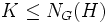# Intermediately isomorph-conjugate subgroup

This article defines a subgroup property: a property that can be evaluated to true/false given a group and a subgroup thereof, invariant under subgroup equivalence. View a complete list of subgroup properties[SHOW MORE]

## Definition

### Symbol-free definition

A subgroup of a group is termed intermediately isomorph-conjugate if it is isomorph-conjugate in every intermediate subgroup.

### Definition with symbols

A subgroup$H$ of a group$G$ is termed intermediately isomorph-conjugate if given any other subgroup$L$ of$G$ which is isomorphic to$H$, there is an element$x \in $ such that$xHx^{-1} = L$.

## Formalisms

BEWARE! This section of the article uses terminology local to the wiki, possibly without giving a full explanation of the terminology used (though efforts have been made to clarify terminology as much as possible within the particular context)

### In terms of the intermediately operator

This property is obtained by applying the intermediately operator to the property: isomorph-conjugate subgroup
View other properties obtained by applying the intermediately operator

## Metaproperties

### Intermediate subgroup condition

YES: This subgroup property satisfies the intermediate subgroup condition: if a subgroup has the property in the whole group, it has the property in every intermediate subgroup.
ABOUT THIS PROPERTY: View variations of this property satisfying intermediate subgroup condition | View variations of this property not satisfying intermediate subgroup condition
ABOUT INTERMEDIATE SUBROUP CONDITION:View all properties satisfying intermediate subgroup condition | View facts about intermediate subgroup condition

If$H$ is intermediately isomorph-conjugate in$G$, then$H$ is also intermediately isomorph-conjugate in any intermediate subgroup. This follows from the definition.

### Normalizing joins

This subgroup property is normalizing join-closed: the join of two subgroups with the property, one of which normalizes the other, also has the property.
View other normalizing join-closed subgroup properties

If$H, K \le G$ are intermediately isomorph-conjugate subgroups, and$K \le N_G(H)$, then the join of subgroups$HK$ is also an intermediately isomorph-conjugate subgroup. Further information: Intermediate isomorph-conjugacy is normalizing join-closed